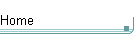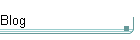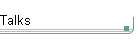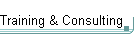GotW #49On theblogNovember 4: Other Concurrency Sessions at PDC November 3 October 26: Hoare on Testing October 23This is the original GotW problem and solution substantially as posted to Usenet. See the book More Exceptional C++ (Addison-Wesley, 2002) for the most current solution to this GotW issue. The solutions in the book have been revised and expanded since their initial appearance in GotW. The book versions also incorporate corrections, new material, and conformance to the final ANSI/ISO C++ standard.

## Template Specialization and OverloadingDifficulty: 6 / 10

How do you specialize and overload templates? When you do, how do you determine which template gets called? Try your hand at analyzing these twelve examples.### Problem

#### JG Questions

1. What is template specialization? Give an example.

2. What is partial specialization? Give an example.

#### Guru Question

3. Consider the following declarations:

```    template<typename T1, typename T2>
void f( T1, T2 );                       // 1
template<typename T> void f( T );       // 2
template<typename T> void f( T, T );    // 3
template<typename T> void f( T* );      // 4
template<typename T> void f( T*, T );   // 5
template<typename T> void f( T, T* );   // 6
template<typename T> void f( int, T* ); // 7
template<> void f<int>( int );          // 8
void f( int, double );                  // 9
void f( int );                          // 10```

Which of the above functions are invoked by each of the following? Be specific by identifying the template parameter types, where appropriate.

```    int             i;
double          d;
float           ff;
complex<double> c;

f( i );         // a
f<int>( i );    // b
f( i, i );      // c
f( c );         // d
f( i, ff );     // e
f( i, d );      // f
f( c, &c );     // g
f( i, &d );     // h
f( &d, d );     // i
f( &d );        // j
f( d, &i );     // k
f( &i, &i );    // l```### Solution

Templates provide C++'s most powerful form of genericity. They allow you to write generic code that works with many kinds of unrelated objects; for example, strings that contain various kinds of characters, containers that can hold arbitrary types of objects, and algorithms that can operate on arbitrary types of sequences.

1. What is template specialization? Give an example.

Template specialization lets templates deal with special cases. Sometimes a generic algorithm can work much more efficiently for a certain kind of sequence (for example, when given random-access iterators), and so it makes sense to specialize it for that case while using the slower but more generic approach for all other cases. Performance is a common reason to specialize, but it's not the only one; for example, you might also specialize a template to work with certain objects that don't conform to the normal interface expected by the generic template.

These special cases can be handled using two forms of template specialization: explicit specialization, and partial specialization.

#### Explicit Specialization

Explicit specialization lets you write a specific implementation for a particular combination of template parameters. For example, given the function template:

`  template<class T> void sort(Array<T>& v) { /*...*/ };`

If we have a faster (or other specialized) way we want to deal specifically with arrays of char*'s, we could explicitly specialize:

`  template<> void sort<char*>(Array<char*>&);`

The compiler will then choose the most appropriate template:

```  Array<int>   ai;
Array<char*> apc;

sort( ai );       // calls sort<int>
sort( apc );      // calls specialized sort<char*>```

#### Partial Specialization

2. What is partial specialization? Give an example.

For class templates only, you can define partial specializations that don't have to fix all of the primary (unspecialized) class template's parameters.

Here is an example from 14.5.4 [temp.class.spec]. The first template is the primary class template:

```  template<class T1, class T2, int I>
class A             { };             // #1```

We can specialize this for the case when T2 is a T1*:

```  template<class T, int I>
class A<T, T*, I>   { };             // #2```

Or for the case when T1 is any pointer:

```  template<class T1, class T2, int I>
class A<T1*, T2, I> { };             // #3```

Or for the case when T1 is int and T2 is any pointer and I is 5:

```  template<class T>
class A<int, T*, 5> { };             // #4```

Or for the case when T2 is any pointer:

```  template<class T1, class T2, int I>
class A<T1, T2*, I> { };             // #5```

Declarations 2 to 5 declare partial specializations of the primary template. The compiler will then choose the appropriate template. From 14.5.4.1 [temp.class.spec.match]:

```  A<int, int, 1>   a1;  // uses #1
A<int, int*, 1>  a2;  // uses #2, T is int,
//          I is 1
A<int, char*, 5> a3;  // uses #4, T is char
A<int, char*, 1> a4;  // uses #5, T1 is int,
//          T2 is char,
//          I is 1
A<int*, int*, 2> a5;  // ambiguous:
// matches #3 and #5```

Now let's consider function template overloading. It isn't the same thing as specialization, but it's related to specialization.

C++ lets you overload functions, yet makes sure the right one is called:

```  int  f( int );
long f( double );

int    i;
double d;

f( i );   // calls f(int)
f( d );   // calls f(double)```

Similarly, you can also overload function templates, which brings us to the final question:

3. Consider the following declarations:

```    template<typename T1, typename T2>
void f( T1, T2 );                       // 1
template<typename T> void f( T );       // 2
template<typename T> void f( T, T );    // 3
template<typename T> void f( T* );      // 4
template<typename T> void f( T*, T );   // 5
template<typename T> void f( T, T* );   // 6
template<typename T> void f( int, T* ); // 7
template<> void f<int>( int );          // 8
void f( int, double );                  // 9
void f( int );                          // 10```

First, let's simplify things a little by noticing that there are two groups of overloaded f's here: Those that take a single parameter, and those that take two parameters. [Note: I deliberately didn't muddy the waters by including an overload with two parameters where the second parameter had a default. Had there been such a function, then for the purposes of determining the correct ordering it should be considered in both lists: once as a single-parameter function (using the default), and once as a two-parameter funtion (not using the default).]

```    template<typename T1, typename T2>
void f( T1, T2 );                       // 1
template<typename T> void f( T, T );    // 3
template<typename T> void f( T*, T );   // 5
template<typename T> void f( T, T* );   // 6
template<typename T> void f( int, T* ); // 7
void f( int, double );                  // 9

template<typename T> void f( T );       // 2
template<typename T> void f( T* );      // 4
template<> void f<int>( int );          // 8
void f( int );                          // 10```

Now let's consider each of the calls in turn:

Which of the above functions are invoked by each of the following? Be specific by identifying the template parameter types, where appropriate.

```    int             i;
double          d;
float           ff;
complex<double> c;

f( i );         // a```

A. This calls #10, because it's an exact match for #10 and such non-templates are always preferred over templates (see 13.3.3).

`    f<int>( i );    // b`

B. This calls #8, because f<int> is being explicitly requested.

`    f( i, i );      // c`

C. This calls #3 (T is int), because that is the best match.

`    f( c );         // d`

D. This calls #2 (T is complex<double>), because no other f can match.

`    f( i, ff );     // e`

E. This calls #1 (T1 is int, T2 is float). You might think that #9 is very close -- and it is -- but a nontemplate function is preferred only if it is an exact match.

`    f( i, d );      // f`

F. This one does call #9, because now #9 is an exact match and the nontemplate is preferred.

`    f( c, &c );     // g`

G. This calls #6 (T is complex<double>), because #6 is the closest overload. #6 provides an overload of f where the second parameter is a pointer to the same type as the first parameter.

`    f( i, &d );     // h`

H. This calls #7 (T is double), because #7 is the closest overload.

`    f( &d, d );     // i`

I. This calls #5 (T is double). #5 provides an overload of f where the first parameter is a pointer to the same type as the second parameter.

Only a few more to go...

`    f( &d );        // j`

J. Clearly (by now), we're asking for #4 (T is double).

`    f( d, &i );     // k`

K. Several other overloads are close, but only #1 fits the bill here (T1 is double, T2 is int*).

And finally...

`    f( &i, &i );    // l`

L. This calls #3 (T is int*), which is the closest overload even though some of the others explicitly mention a pointer parameter.

The Good News:

Compiler vendors will soon be supporting templates better, so that you can make use of features like the above more reliably and portably.